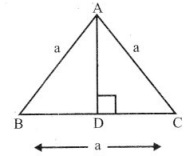# Grade 7 The Triangle and Its Properties Worksheets

### Grade 7 Maths The Triangle and Its Properties Multiple Choice Questions (MCQs)

1. How many medians a triangle can have?
(a) 2
(b) 1
(c) 3
(d) 0

2. A/an …………….. connect a vertex of a triangle to the mid point of the opposite side.
(a) altitude
(b) median
(c) vertex
(d) none of these

3. How many altitude can a triangle have?
(a) 1
(b) 2
(c) 3
(d) 4

4. Find the value of x in the adjoining figure.
(a) 50°
(b) 70°
(c) 120°
(d) 180°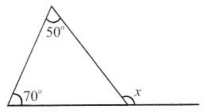5. Find the value of x
(a) 60°
(b) 110°
(c) 50°
(d) 180°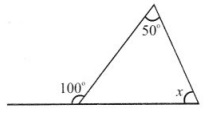6. A triangle in which two sides are of equal lengths is called ………………….. .
(a) Equilateral
(b) Isosceles
(c) Scalene
(d) Acute angled triangle

7. In the Pythagoras property, the triangle must be …………….. .
(a) acute angled
(b) right angled
(c) obtuse angled
(d) none of these

8. Find the value of x in this figure.
(a) 40°
(b) 60°
(c) 35°
(d) 180°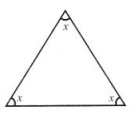9. Find the value’of x in given figure.
(a) 180°
(b) 55°
(c) 90°
(d) 60°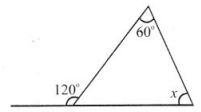10. A triangle in which all three sides are of equal lengths is called ……………. .
(a) Equilateral
(b) Isosceles
(c) Scalene
(d) Acute angled triangle

11. ∆ ABC is right-angled at C. If AC = 5 cm and BC = 12 cm find the length of AB.
(a) 7 cm
(b) 17cm
(c) 13 cm
(d) none of these

12. PQR is a triangle right angled at P. If PQ = 3 cm and PR = 4 cm, find QR.
(a) 7 cm
(b) 17 cm
(c) 13 cm
(d) none of these.

13. Which is the longest side in the triangle PQR right angled at P?
(a) PQ
(b) QR
(c) PR
(d) none of these.

14. Which is the longest side in the triangle ABC right angled at B?
(a) AB
(b) BC
(c) AC
(d) none of these.

15. Which is the longest side of a right triangle?
(a) perpendicular
(b) base
(c) hypotenuse
(d) none of these.

16. In a ∆ ABC, ∠A = 35° and ∠B = 65°, then the measure of ∠C is:
(a) 50°
(b) 80°
(c) 30°
(d) 60°

17. The hypotenuse of a right triangle is 17 cm long. If one of the remaining two sides is 8 cm in length, then the length of the other side is:
(a) 15 cm
(b) 12 cm
(c) 13 cm
(d) none of these.

18. How many acute angles can a right triangle have?
(a) 1
(b) 2
(c) 3
(d) 0

19. The acute angles of right triangle are in the ratio 2 : 1. Find the measure of each of these angles.
(a) 55° and 35°
(b) 60° and 30°
(c) 50° and 40°
(d) 45° and 45°

20. One of the angles of a triangle is 100* and the other two angles are equal. Find the measure of each of these equal angles.
(a) 45°
(b) 40°
(c) 41°
(d) 42°

### Grade 7 Maths Lines and Angles Fill In The Blanks

1. Every triangle has at least ……………. acute angles.
2. The longest side of a right angled triangle is called its …………….. .
3. Median is also called …………….. in an equilateral triangle.
4. The line segment joining a vertex of a triangle to the mid-point of its opposite side is called its ……….. .
5. Measures of each of the angles of an equilateral triangle is ……………… .

### Grade 7 Maths Lines and Angles True(T) And False(F)

1. Sum of two sides of a triangle is greater than or equal to the third side.
2. The difference between the lengths of any two sides of a triangle is smaller than the length of third side.
3. Sum of any two angles of a triangle is always greater than the third angle.
4. It is possible to have a right angled equilateral triangle.
5. It is possible to have a triangle in which two of the angles are right angles.

### Grade 7 Maths Lines and Angles Very Short Answer Type Questions

Find the value of x:

1.2.3.4.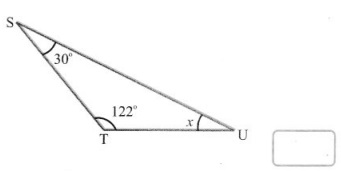5. If one angle of a triangle is 60° and the other two angles are in the ratio 1 : 2, then find the angles.
6. In figure find the value of ∠A + ∠B + ∠C + ∠D + ∠E + ∠F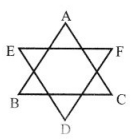Find the value of x an y:

1. Here CD || AB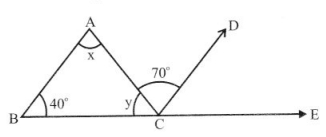2.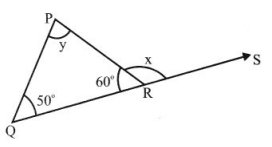3.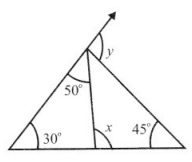4.